Question

The mean price for a 2,000sq foot home in FL is $240,000 with a Standard Deviation of$16,000. The mean price of the same sized home in OH is $170,000 with a standard deviation of$12,000. Which state would a home priced at $200,000 be closer to the mean price, compared to the distribution of prices in the state? Find the z score for each state. in progress 0 1 month 2021-08-12T07:58:27+00:00 1 Answers 1 views 0 ## Answers ( ) 1. Answer: The z-score for a home priced at$200,000 in Florida is of -2.5.

The z-score for a home priced at $200,000 in Ohio is of 2.5. The closeness to the mean is measured by the absolute z-score(disconsidering the signal, the lower the score, the closer to the mean). However, in this case, both z-scores have the same absolute value, so in both Florida and Ohio a home priced at$200,000 is equally close to the mean.

Step-by-step explanation:

Z-score:

In a set with mean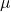and standard deviation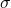, the z-score of a measure X is given by: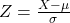The Z-score measures how many standard deviations the measure is from the mean. After finding the Z-score, we look at the z-score table and find the p-value associated with this z-score. This p-value is the probability that the value of the measure is smaller than X, that is, the percentile of X. Subtracting 1 by the p-value, we get the probability that the value of the measure is greater than X.

The mean price for a 2,000sq foot home in FL is $240,000 with a Standard Deviation of$16,000. Home of $200,000. This means that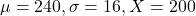. So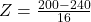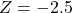The z-score for a home priced at$200,000 in Florida is of -2.5.

The mean price of the same sized home in OH is $170,000 with a standard deviation of$12,000. Home of $200,000. This means that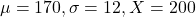. So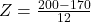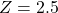The z-score for a home priced at$200,000 in Ohio is of 2.5.

Which state would a home priced at $200,000 be closer to the mean price, compared to the distribution of prices in the state? The closeness to the mean is measured by the absolute z-score(disconsidering the signal, the lower the score, the closer to the mean). However, in this case, both z-scores have the same absolute value, so in both Florida and Ohio a home priced at$200,000 is equally close to the mean.